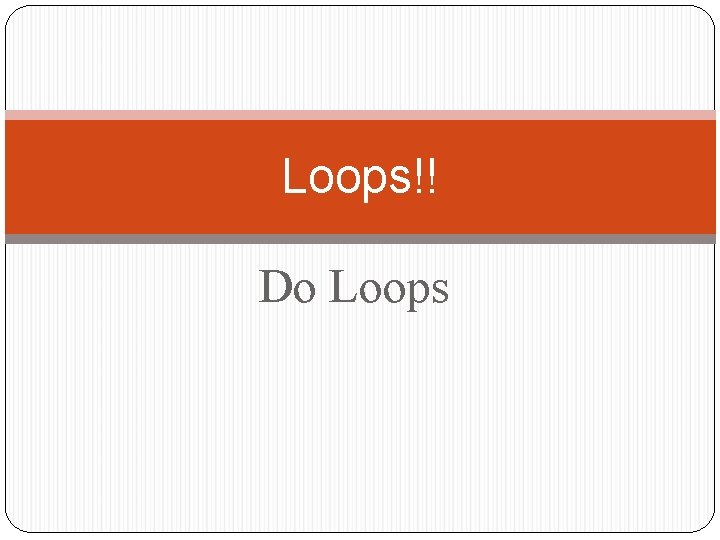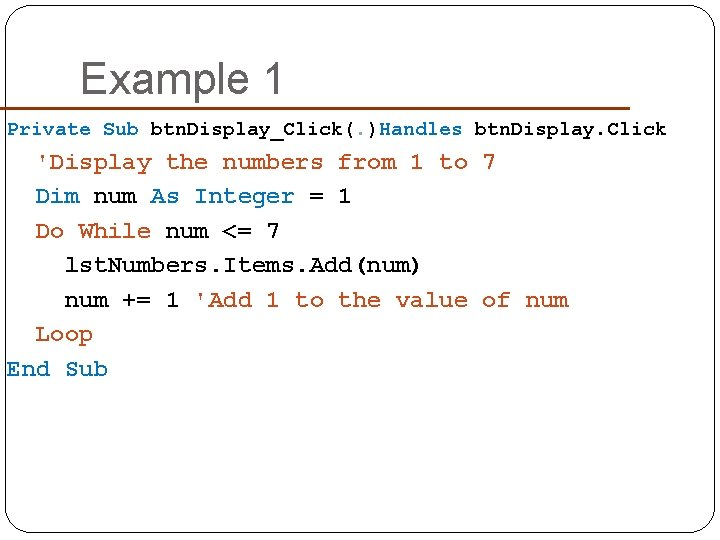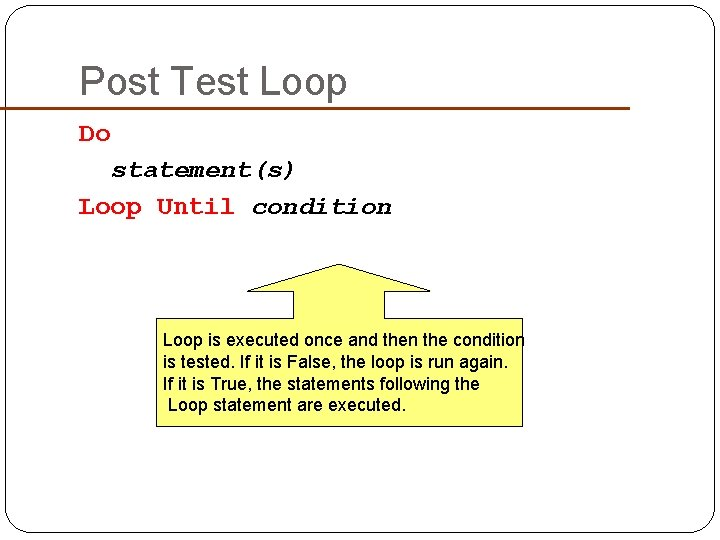# Loops Do Loops Do Loops A loop is

• Slides: 9Loops!! Do LoopsDo Loops A loop is one of the most important structures in programming. Used to repeat a sequence of statements a number of times. The Do loop repeats a sequence of statements either as long as or until a certain condition is true.Do Loop Syntax Do While condition statement(s) Loop These statements are inside the body of the loop and are run if the condition above is True. Condition is tested, If it is True, the loop is run. If it is False, the statements following the Loop statement are executed.Example 1 Private Sub btn. Display_Click(. )Handles btn. Display. Click 'Display the numbers from 1 to 7 Dim num As Integer = 1 Do While num <= 7 lst. Numbers. Items. Add(num) num += 1 'Add 1 to the value of num Loop End SubExample: Repeat Request as Long as Response is Incorrect Dim pass. Word As String = "“ Do While pass. Word <> "SHAZAM“ pass. Word = Input. Box("What is the password? ") pass. Word = pass. Word. To. Upper Loop pass. Word is the loop control variable because the value stored in pass. Word is what is tested to determine if the loop should continue or stop.Example 4: Write a program which allows you to deposit money into a savings account that accumulates 6% interest compounded annually and determines when you are a millioniare. txt. Amount txt. WhenPrivate Sub btn. Calculate_Click(. . . ) Handles btn. Calculate. Click Dim balance As Double, num. Years As Integer balance = CDbl(txt. Amount. Text) Do While balance < 1000000 balance += 0. 06 * balance num. Years += 1 Loop txt. When. Text = "In " & num. Years & _ " years you will have a million dollars. " End SubPost Test Loop Do statement(s) Loop Until condition Loop is executed once and then the condition is tested. If it is False, the loop is run again. If it is True, the statements following the Loop statement are executed.Example: Do pass. Word = Input. Box("What is the pass. Word = pass. Word. To. Upper Loop Until pass. Word = "SHAZAM" password? ")